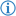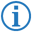The not equal operator is a type of comparison operator which returns the Boolean value `false` if and only if the given objects are equal, else it returns `true`.

Its token is named NOT_EQUAL.

## FDS### Example

`If`` ``Spike's age`` ``was not`` ``10`` ``then``:` `...`

This executes the next part of code only if the variable `Spike's age` is different from `10`.

This is equivalent to the following Java code:

```if(Spike_s_age!=10) { ...
```